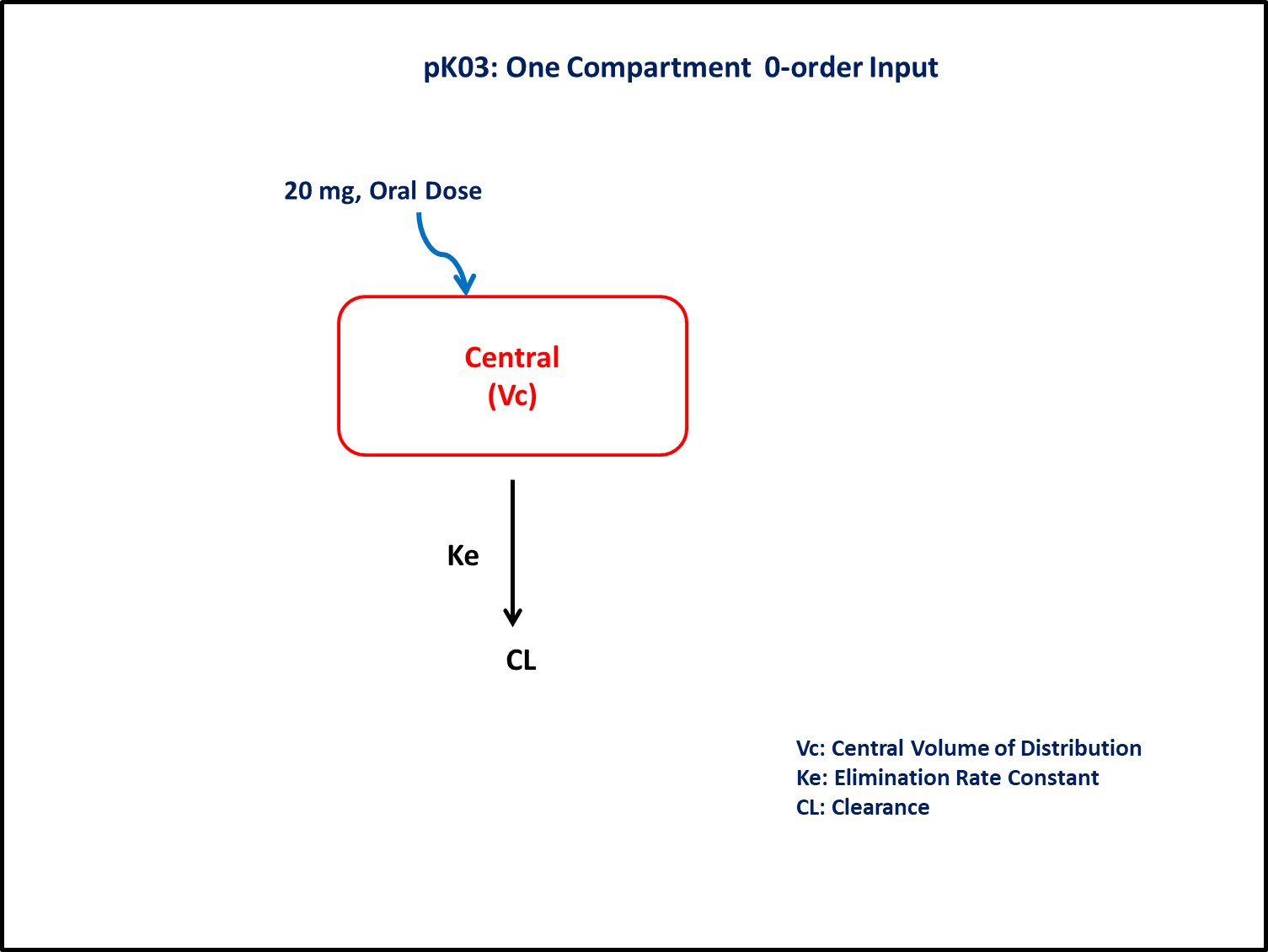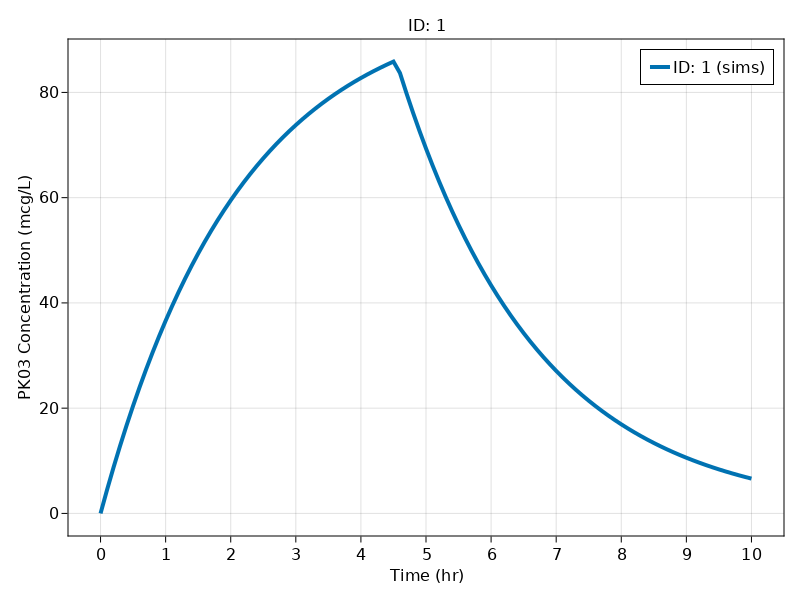# Exercise PK03 - One Compartment 1ˢᵗ- and 0-order input

### Background

Following info:

• Structural model - One compartment linear elimination with zero-order absorption

• Route of administration - Oral

• Dosage Regimen - 20 mg Oral

• Number of Subjects - 1In this model, collection of plasma concentration data, will help you to derive/estimate the parameters: Clearance, Volume of Distribution, Duration of zero-order input.

### Objectives

In this tutorial, you will learn how to build one compartment model for zero-order input and simulate the model for a single subject.

### Libraries

call the "necessary" libraries to get started.

using Random
using Pumas
using PumasUtilities
using CairoMakie


### Model

In this one compartment model, we administer dose in Central compartment as a zero-order input and estimate the rate of input.

pk_03        = @model begin
desc = "One Compartment Model with zero-order input"
timeu = u"hr"
end

@param begin
"Clearance (L/hr)"
tvcl     ∈ RealDomain(lower=0)
"Volume (L)"
tvvc     ∈ RealDomain(lower=0)
"Assumed Duration of Zero-order (hr)"
tvTabs   ∈ RealDomain(lower=0)
Ω        ∈ PDiagDomain(3)
"Proportional RUV"
σ²_prop  ∈ RealDomain(lower=0)
end

@random begin
η        ~ MvNormal(Ω)
end

@pre begin
Cl       = tvcl * exp(η)
Vc       = tvvc * exp(η)
end

@dosecontrol begin
duration = (Central = tvTabs * exp(η),)
end

@dynamics begin
Central' =  - (Cl/Vc)*Central
end

@derived begin
"""
PK03 Concentration (mcg/L)
"""
cp       = @. 1000*(Central/Vc)
"""
PK03 Concentration (mcg/L)
"""
dv       ~ @. Normal(cp, sqrt(cp^2*σ²_prop))
end
end

PumasModel
Parameters: tvcl, tvvc, tvTabs, Ω, σ²_prop
Random effects: η
Covariates:
Dynamical variables: Central
Derived: cp, dv
Observed: cp, dv


### Parameters

• $Cl$ - Clearance (L/hr)

• $Vc$ - Volume of Central Compartment (L)

• $Tabs$ - Assumed duration of zero-order input (hrs)

• $Ω$ - Between Subject Variability

• $σ$ - Residual error

param = ( tvcl     = 45.12,
tvvc     = 96,
tvTabs   = 4.54,
Ω        = Diagonal([0.0,0.0,0.0]),
σ²_prop  = 0.015)

(tvcl = 45.12, tvvc = 96, tvTabs = 4.54, Ω = [0.0 0.0 0.0; 0.0 0.0 0.0; 0.0
0.0 0.0], σ²_prop = 0.015)


### Dosage Regimen

Single 20 mg or 20000μg Oral dose given to a subject.

Note:- In this the dose administered is on mg and conc are in μg/L, hence a scaling factor of 1000 is used in the @derived block in the model.

ev1   = DosageRegimen(20, rate = -2)
sub  = Subject(id = 1, events = ev1, observations = (cp = nothing,))

Subject
ID: 1
Events: 2
Observations: cp: (nothing)


### Simulation

Lets simulate for plasma concentration with the specific observation time points after oral administration.

Random.seed!(123)
sim = simobs(pk_03, sub, param, obstimes = 0:0.1:10)


### Visualize results

f, a, p = sim_plot(pk_03, [sim], observations=:cp,
linewidth = 4,
axis = (ylabel = "PK03 Concentration (mcg/L)",
xticks = 0:1:10,)
)
axislegend(a)
f